# Cubic equation

(diff) ← Older revision | Latest revision (diff) | Newer revision → (diff)

An algebraic equation of degree three, i.e. an equation of the formwhere. Replacingin this equation by the new unknowndefined by, one brings the equation to the following simpler (canonical) form:where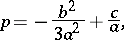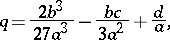and the solution to this equation may be obtained by using Cardano's formula (cf. Cardano formula); in other words, any cubic equation is solvable in radicals.

The cubic equation was first solved in the 16th century. At the beginning of that century, S. Ferro solved the equation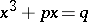, where,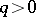, but did not publish his solution. N. Tartaglia rediscovered Ferro's result; he also solved the equation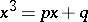(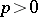,), and announced without proof that the equation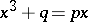(,) could be reduced to that form. Tartaglia communicated his results to G. Cardano, who published the solution of the general cubic equation in 1545.

How to Cite This Entry:
Cubic equation. Encyclopedia of Mathematics. URL: http://encyclopediaofmath.org/index.php?title=Cubic_equation&oldid=15586
This article was adapted from an original article by I.V. Proskuryakov (originator), which appeared in Encyclopedia of Mathematics - ISBN 1402006098. See original article